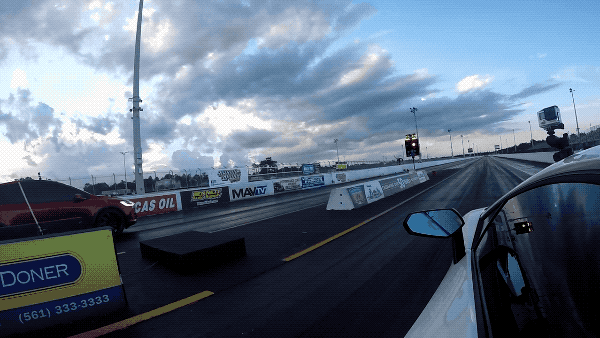## 20170922

### Physics quiz question: Tesla Model X P100D drag race acceleration

Physics 205A Quiz 2, fall semester 2017
Cuesta College, San Luis Obispo, CA"Tesla Model X P100D Ludicrous..."
DragTimes
youtu.be/_NnNEuxqoPo

A Tesla Model X P100D took 10.947 s to travel 402 m, speeding up with constant acceleration after starting from rest. Assume that car traveled along a straight horizontal line, and that the acceleration was always pointed in the same direction as the velocity. The magnitude of the acceleration of the car was:
(A) 3.4 m/s2.
(B) 6.7 m/s2.
(C) 37 m/s2.
(D) 73 m/s2.

[*] dragtimes.com/Tesla-Model-X-Timeslip-29390.html.

Correct answer (highlight to unhide): (B)

The following quantities are given (or assumed to be known):

(x0 = 0 m),
(t0 = 0 s),
x = +402 m,
t = 10.947 s,
v0x = 0 m/s.

So in the equations for constant (average) acceleration motion in the horizontal direction, the following quantities are unknown, or are to be explicitly solved for:

vx = v0x + ax·t,

x = (1/2)·(vx + v0xt,

x = v0x·t + (1/2)·ax·(t)2,

vx2 = v0x2 + 2·ax·x.

With the unknown quantity ax to be solved for appearing in the third equation, with all other quantities given (or assumed to be known), then:

x = v0x·t + (1/2)·ax·(t)2,

+402 m = (0 m/s)·(10.947 s) + (1/2)·ax·(10.947 s)2,

+402 m = 0 + ax·(59.9184045 s2),

(+402 m)/(59.9184045 s2) = ax = +6.7091239053 m/s2,

which to two significant figures is +6.7 m/s2.

(Response (A) is x/(t2); response (C) is x/t; response (D) is 2·x/t.)

Sections 70854, 70855
Exam code: quiz02BjRn
(A) : 5 students
(B) : 40 students
(C) : 4 students
(D) : 6 students

Success level: 73%
Discrimination index (Aubrecht & Aubrecht, 1983): 0.64